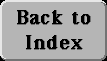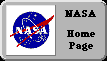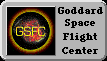# (M-3) Formulas

Unknown numbers are usually represented by letters of the alphabet. The letterx is the all-out favorite, with y used when a second unknown is needed and z for a third. By the way, the name used for the unknown number by early mathematicians (like Al-Khorezmi) was "the thing"--"shai" in Arabic, "res" in the Latin which scholars used in Europe.

However, different letters are also used, often hinting at the quantity they represent--v for an unknown velocity, T for an unknown time,m or M for unknown masses and F for an unknown force. (These will be used later in the calculation of Lagrangian points).

"Unknown" may be broadened to include "unspecified"--letters standing for numbers which may actually be known, but with whose exact values we do not want to bother, either because (#1) we want to save writing them out, or (#2) because we have not yet chosen them.

An example of case (#1) is the ratio between the length of a circle and its diameter, a universal constant appearing in many equations (for example, Kepler's equation in section (12a)), whose value to 11 decimals is 3.14159265359... Although the number is known, it is universally represented in all equations by the letter p (Greek p, pronounced "pi"). It is only replaced with the actual number when the unknown quantity is derived.

As an example of case (#2), consider the distance s which a dropped ball covers in a time of t seconds, starting from rest. It is

s = (1/ 2) gt2

(touching symbols--including the term (1/ 2)--get multiplied, remember?).
Here g is the number giving the strength of the Earth's gravity pull: if s is in meters, g = 9.81, if in feet, g = 32.16 (9.81 meters = 32.16 feet). It is known, but (as in example #1 above), you don't bother with its actual value until the moment you actually use it. But the time t is not yet chosen! Whatever you choose for t, the formula will give you the appropriate distances.

The above is a typical formula. It looks like an equation, but the game is a little different: you are not asked to "dig out the unknown number," because it is already in plain sight. All you do is choose the value of t and you get the corresponding value of s.

Still, the "digging out" skills you learn with equations are also useful here. Suppose you seek the inverse relationship--given s, what is t? One now views t as the unknown and proceeds to isolate it. Multiply both sides by 2

2s = gt2

and divide by g

2s/ g = t2

To go from t2 to t one must find the square root, an easy task for anyone with a calculator having a square root button (slower methods also exist, using pencil and paper). Mathematics has a sign for this, but since the web does not provide it, we write instead SQRT:

SQRT(2s/ g) = t

Now, whatever the distance s is, one can put it in the equation and derive the appropriate time t, in seconds.

Next Stop:   #M-4    IdentitiesAuthor and curator: David P. Stern, u5dps@lepvax.gsfc.nasa.gov

Last updated 25 February 1999